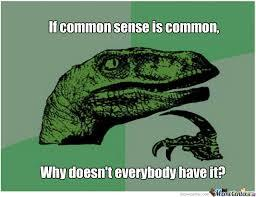End of the year
3 years ago
lisaklemens
Save
Edit
Host a game
Live GameLive
Homework
Solo Practice
Practice15 QuestionsShow answers
• Question 1
30 seconds
Q. A farmer has 13 cows. A bolt of lightning kills all but 5 of them. How many cows survived?
answer choices
0
8
5
• Question 2
30 seconds
Q. Your computer is loading. It tells you to press any key. What do you do?
answer choices
Look for the "any" key
Press any button you want
Press "A", "N" and "Y" at the same time
• Question 3
30 seconds
Q. Which is greater: six dozen dozen or half a dozen dozen?
answer choices
6 dozen dozen
1/2 dozen dozen
Both the same
• Question 4
30 seconds
Q. How many months are in a decade?
answer choices
10
120
12
• Question 5
30 seconds
Q. How many times you can subtract the number 5 from 25?
answer choices
Once
Five
Eight
• Question 6
30 seconds
Q. The day before yesterday, Suzie was 17. Next year, she will be 20. What day is her birthday?
answer choices
Jan 1st
December 31st
None of the above
• Question 7
30 seconds
Q. If you were to put a coin into an empty bottle and then insert a cork into the neck, how could you remove the coin without taking out the cork or breaking the bottle?
answer choices
Use a cork remover to remove the cork
Twist the cork off
Push the cork ito the bottle and shake the coin out
• Question 8
30 seconds
Q. Start with 100. Add 10. Add 100. Add 20. Add 100. Add 30. Add 100. Add 40.
answer choices
300
410
500
• Question 9
30 seconds
Q. Jordan has 3 coins equaling 55 cents and one is not a nickel. What are the 3 coins?
answer choices
2 quarters and a nickel
impossible
Half dollar with some pennies
• Question 10
30 seconds
Q. What occurs twice in a week, once in a year, but never in a day?
answer choices
e
a day that starts with S
Leap Day
Dancing with the Stars
• Question 11
30 secondsQ.
answer choices
a
b
c
d
• Question 12
30 secondsQ.
answer choices
Four get Ten Heros
Get Heros
Forgotten Heros
Ten Heros
• Question 13
30 secondsQ. What is the value of the question mark?
answer choices
5
54
94
18
• Question 14
30 secondsQ. What spot number is the red car parked in?
answer choices
98
87
89
72
• Question 15
30 secondsQ. What is the value of the question mark?
answer choices
43
44
11
9
Report Quiz
Why show ads?
Report Ad